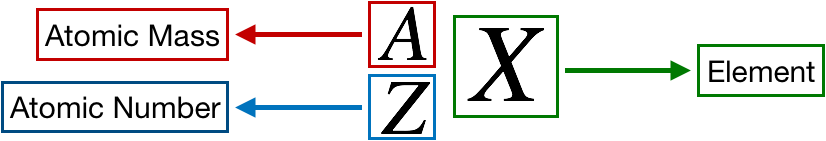# Problem: An atom of oxygen has an atomic number of 8 and a mass number of 18. How many of each type of subatomic particle does it contain?

###### FREE Expert Solution

We’re being asked to determine the number of protons, electrons, and neutrons in oxygen, O-18

Recall that the symbol for an element or ion is as follows:86% (283 ratings)###### Problem Details

An atom of oxygen has an atomic number of 8 and a mass number of 18. How many of each type of subatomic particle does it contain?